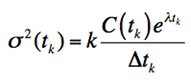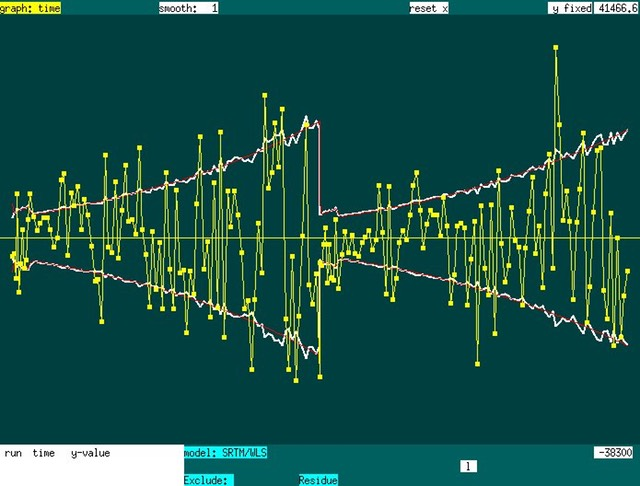## Noise models

If one omits the keyword “half-life” in the SRTM model file, then the program has no idea about what isotope [11C, 18F] was used for the PET run. In this case, the analysis will assume equal weights for all time points, so the analysis uses ordinary least squares (OLS). In fact, the weighting is not quite OLS, because it still assumes that the variance scales inversely with the length of PET frames, but otherwise there is no time dependence.

If a decay time is specified (e.g., “half-life 20.33” for the 11C half-life in minutes), then a standard PET noise model is used, and analysis employs weighted least squares (WLS). The noise model is a standard one that assumes that the variance scales with magnitude of the signal, which has been converted to a concentration (C) by decay-correcting the data using the known half-life, and that bin size also matters:The program then uses a diagonal variance matrix and solves the linear equations using standard WLS.

Below: Residue of fit (yellow points) for a double-bolus experiment (two 11C syntheses), together with the raw noise model  (white lines) dependent upon signal intensities using the formula above, and a regularized noise model (red curve) fit by polynomials. The WLS weighting matrix employs the polynomial-regularized (red) variance model.Joseph B. Mandeville, Athinoula A. Martinos Center for Biomedical Imaging at MGH/MIT/Harvard